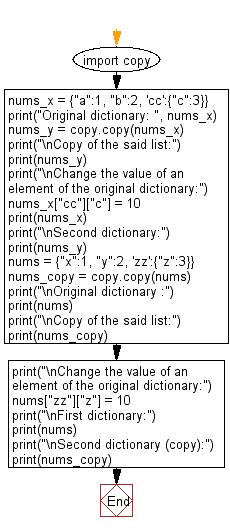﻿ Python: Create a shallow copy of a given dictionary - w3resource# Python: Create a shallow copy of a given dictionary

## Python module: Exercise-3 with Solution

Write a Python program to create a shallow copy of a given dictionary. Use copy.copy

Sample Solution:

Python Code:

``````import copy
nums_x = {"a":1, "b":2, 'cc':{"c":3}}
print("Original dictionary: ", nums_x)
nums_y = copy.copy(nums_x)
print("\nCopy of the said list:")
print(nums_y)
print("\nChange the value of an element of the original dictionary:")
nums_x["cc"]["c"] = 10
print(nums_x)
print("\nSecond dictionary:")
print(nums_y)

nums = {"x":1, "y":2, 'zz':{"z":3}}
nums_copy = copy.copy(nums)
print("\nOriginal dictionary :")
print(nums)
print("\nCopy of the said list:")
print(nums_copy)
print("\nChange the value of an element of the original dictionary:")
nums["zz"]["z"] = 10
print("\nFirst dictionary:")
print(nums)
print("\nSecond dictionary (copy):")
print(nums_copy)
```
```

Sample Output:

```Original dictionary:  {'a': 1, 'b': 2, 'cc': {'c': 3}}

Copy of the said list:
{'a': 1, 'b': 2, 'cc': {'c': 3}}

Change the value of an element of the original dictionary:
{'a': 1, 'b': 2, 'cc': {'c': 10}}

Second dictionary:
{'a': 1, 'b': 2, 'cc': {'c': 10}}

Original dictionary :
{'x': 1, 'y': 2, 'zz': {'z': 3}}

Copy of the said list:
{'x': 1, 'y': 2, 'zz': {'z': 3}}

Change the value of an element of the original dictionary:

First dictionary:
{'x': 1, 'y': 2, 'zz': {'z': 10}}

Second dictionary (copy):
{'x': 1, 'y': 2, 'zz': {'z': 10}}
```

Flowchart:## Visualize Python code execution:

The following tool visualize what the computer is doing step-by-step as it executes the said program:

Python Code Editor:

Have another way to solve this solution? Contribute your code (and comments) through Disqus.

What is the difficulty level of this exercise?

Test your Python skills with w3resource's quiz

﻿

## Python: Tips of the Day

For-else construct useful when searched for something and find it:

```# For example assume that I need to search through a list and process each item until a flag item is found and
# then stop processing. If the flag item is missing then an exception needs to be raised.

for i in mylist:
if i == theflag:
break
process(i)
else:
raise ValueError("List argument missing terminal flag.")
```